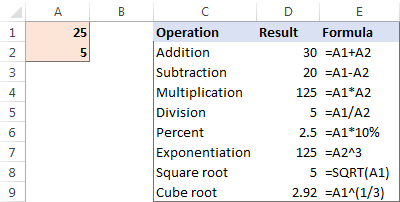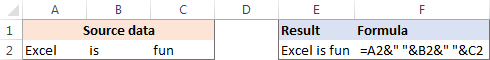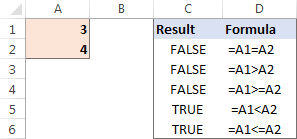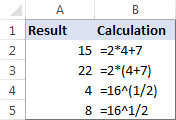# How to do calculations in Excel

The tutorial shows how to do arithmetic calculations in Excel and change the order of operations in your formulas.

When it comes to calculations, there is almost noting that Microsoft Excel cannot do, from totaling a column of numbers to solving complex linear programming problems. For this, Excel provides a few hundred predefined formulas, called Excel functions. In addition, you can use Excel as a calculator to do math - add, divide, multiply, and subtract numbers as well as raise to power and find roots.

## How to do calculations in Excel

Making calculations in Excel is easy. Here's how:

• Type the equal symbol (=) in a cell. This tells Excel that you are entering a formula, not just numbers.
• Type the equation you want to calculate. For example, to add up 5 and 7, you type =5+7
• Press the Enter key to complete your calculation. Done!

Instead of entering numbers directly in your calculation formula, you can put them in separate cells, and then reference those cells in your formula, e.g. =A1+A2+A3

The following table shows how to perform basic arithmetic calculations in Excel.

 Operation Operator Example Description Addition + (plus sign) =A1+A2 Adds up the numbers in cells A1 and A2. Subtraction - (minus sign) =A1-A2 Subtracts the number in A2 from the number in A1. Multiplication * (asterisk) =A1*A2 Multiplies the numbers in A1 and A2. Division / (forward slash) =A1/A2 Divides the number in A1 by the number in A2. Percent % (percent) =A1*10% Finds 10% of the number in A1. Raising to power (Exponentiation) ^ (caret) =A2^3 Raises the number in A2 to the power of 3. Square root SQRT function =SQRT(A1) Finds the square root of the number in A1. Nth root ^(1/n) (Where n is the root to find) =A1^(1/3) Finds the cube root of the number in A1.

The results of the above Excel calculation formulas may look something similar to this:Apart from that, you can combine values from two or more cells in a single cell by using the concatenation operator (&) like this:

`=A2&" "&B2&" "&C2`

A space character (" ") is concatenated in between cells to separate the words:You can also compare cells by using logical operators such as "greater than" (>), "less than" (<), "greater than or equal to" (>=), and "less than or equal to" (<=). The result of comparison are logical values of TRUE and FALSE:## The order in which Excel calculations are performed

When you do two or more calculations in a single formula, Microsoft Excel calculates the formula from left to right, according to the order of operations shown in this table:

 Precedence Operation 1 Negation, i.e. reversing the number sign, as in -5, or -A1 2 Percent (%) 3 Exponentiation, i.e. raising to power (^) 4 Multiplication (*) and division (/), whichever comes first 5 Addition (+) and subtraction (-), whichever comes first 6 Concatenation (&) 7 Comparison (>, <, >=, <=, =)

Since the order of calculations affects the final result, you need to know how to change it.

## How to change the order of calculations in Excel

Like you do in math, you can change the order of Excel calculations by enclosing the part to be calculated first in parentheses.

For example, the calculation `=2*4+7` tells Excel to multiply 2 by 4, and then add 7 to the product. The result of this calculation is 15. By enclosing the addition operation in parentheses `=2*(4+7)`, you instruct Excel to add up 4 and 7 first, and then multiply the sum by 2. And the result of this calculation is 22.

Another example is finding a root in Excel. To get the square root of, say, 16, you can use either this formula:

`=SQRT(16)`

or an exponent of 1/2:

`=16^(1/2)`

Technically, the above equation tells Excel to raise 16 to the power of 1/2. But why do we enclose 1/2 in parentheses? Because if we don't, Excel will raise 16 to the power of 1 first (an exponent operation is performed before division), and then divide the result by 2. Since any number raised to the power of 1 is the number itself, we would end up dividing 16 by 2. In contrast, by enclosing 1/2 in parentheses you tell Excel to divide 1 by 2 first, and then raise 16 to the power of 0.5.

As you can see in the screenshot below, the same calculation with and without parentheses produces different results:This is how you make calculations in Excel. I thank you for reading and hope to see you on our blog next week!

## You may also be interested in

1. Thanks very very import daily work any where.

2. How do i use this to solve thermodynamics calculations

3. Formula d11 it is 30.8the multiply to the 2.83 and add value in cell D 19 (16)

4. =(28.5-24)+(18.5-15) /2 = 3.5

hello,

how would i do this. This is an example of numbers that i will need to calculate

• Hi!
Firstly, you can only convert text to a formula using a VBA macro. Secondly, the result of your expression 3.5 is incorrectly calculated.

5. can use excel to calculate money or do basic account.
thanks

6. Hi,
How do you do this
A/B =12.3
X=12
Y=0.3

THANKS

8. Greetings to u all;
Pls help me out, am Familiar with MS Excel but am still having a little challenge with it. The calculation aspect...pls I need assistance...!

Pls with all due of respect; Add me on WhatsApp...! 08134882649

9. Hi there,
Im trying to excel to pick single or multiple values from a calculation and place them into certain rows/column's. For example excel has calculated a number 2.56 i need it to pick numbers from certain column's

65 10.03
80 7.4
100 2.4
So 2.56 is greater than 2.4 so I need it to grab 80 and 65 and place them into a another column..I hope this makes sense and is this possible

• Hello!
The formula below will do the trick for you:

=FILTER(A1:A4,(B1:B4=SMALL(FILTER(B1:B4,B1:B4>\$B\$8),1)) + (B1:B4=SMALL(FILTER(B1:B4,B1:B4>\$B\$8),2)))

Cell B8 = 2.56

Example:
Sulop M.S 75 (23.50) 36 (25) : What is the sum total of this column? Before adding all column it should be multiply for their each price.
DJ Sean 38 (23.50) 72 (25)

Thank you,

• Hello!
Where is the price here? Are these numbers in the column? What do they mean and what do you want to calculate? Please describe your problem in more detail.

11. Hi, how do you formulate a cell to pull information from another cell but it needs to Show 1 as 5, 2 as 4, 3 as 3, 4 as 2 and 5 as 1?

is there a formula from this?

• Hello!
I recommend using the CHOOSE function.

=CHOOSE(A1,5,4,3,2,1)

12. My wookbook is no longer calculate automatically because I add extra column, please assist on how I'm going to fix this calculation situation

• Hi,
This tutorial explains the basics of Excel calculation settings and how to recalculate Excel formulas automatically and manually.

13. Calculate pensions at 9% ..
In wages ..

14. Hi. Is there a formula to count how many times a certain number appears in a column? I have a spreadsheet with 0, 1, 2, and 3s in each column. I have to manually count how many 1s, 2s, and 3s and enter it into a different table to create graphs. It's painstaking work. Is there a formula instead to do the calculation? Thank you

• Hello!
Solve your problem with the COUNTIF function. Read more in this guide.

15. 125 minus 45.98 minus 7.98 multiple 10000

16. Hello!

How I can sum only the number from cells like this FR - 1?

17. I need to create a formula in a cell that changes based on the value in another cell.

For example, if the value in cell R2 is "E" i want to calculate 8.5% of cell I2 and display this in cell S2 AND if R2 is 'N' I want to calculate 13.5% of cell I2 and display this in cell S2 instead

Is this possible, and if so can someone please provide the formula?

18. Hi, I need to know that which formula i can put for my attendance report for On Time, Late inn, Early Out, Absent. Please help me out for this.

• Hi,
Your task is not completely clear to me. Without seeing your data it is difficult to give you any advice. Please describe your problem in more detail. Provide me with an example of the source data and the expected result. It’ll help me understand it better and find a solution for you.

19. hi I would like to know how to calculate on excel and learning ad doing = but it show B4:bc what should I do them thanks

20. how to compute or solution in this equation in excel 15^5*3+10+2(6)=? thank u.

21. Thank you. Is possible to add an equal sign before a value in excel automatically?

22. Thx. And now how to hide = in cell A1?

23. Hello. How I can make in one cell to stay this 2+2*3 and in other cell result of this?

• Hello!
Write your formula =2+2*3 in cell A2. In cell A1 use the function =FORMULATEXT(A2)

24. thanks but seriously I don't get it all I need more explanation from the scratch

25. how do I use a formula on a spreadsheet on a number of entries without having to enter it each time

26. I have to calculate (11/6) to the power 54. How can I do this on excel? I cannot find the caret function on excel.

• Hello!

=(11/6)^54

The ^ character is at 6 using SHIFT

27. Hello,

Total discount to distributor 42%, they have margin of 9%, what will be the % discount that end customer receives?

28. Excel spread sheet, why when making an percentage formular calculation does it show a negative amount?

• Hello!
If you count the percentage of a negative number, the result will also be a negative number.

29. Hello,
I have excel directories to track test conducted at each location. The directory has columns that state positive or negative with result testing. How do I create a summary page that will calculate the positive and negative findings?

Thank you,
Rhonda

• Hello!
Unfortunately, without seeing your data it hard to give you advice. I recommend you a guide to working with pivot tables. If you have more specific questions and can give an example of the initial data and the desired result, I will try to help you.

30. Hi, I need to calculate the Inductance formula. The Reactance (XL) is in cell K56 and f=60. The calculation is XL/2PIf. I have tried ((K56)/(2*pi*60)) and variations of this and cannot get it correct. Can you help?

31. Question.. I want to have this formula in a cell (200)/(1+x/100)=n, I want the formula to be invisible in the box and only put out a number (n) when I type in a value for x in the formula cell, thanks

• Hello Matthew!

=IF(A1 <> "",(200)/(1+A1/100),"")

The formula will show the value only if cell A1 is not empty

32. Hi,
If I would like to multiply (10000 x 8%) ÷12. Is the formula (10000*8%)/12? I can't seem to get it right on excel. And also if I want to calculate (10000x8%) x 2 then ÷ 24, What will be the formula like? Appreciate your time and thank you in advance!

• formula would be =(10000*.08)/12

33. No. Salary Range New Tax Rate. Deduction
1. 0 – 600 Birr. Non-Taxable –
2 601 – 1,650 Birr 10%. 60 Birr
3. 1,651 – 3,200 Birr. 15% 142.50 Birr
4. 3,201 – 5,250 Birr. 20% 302.50 Birr
5. 5,251 – 7,800 Birr. 25%. 565 Birr
6. 7,801 – 10,900 Birr 30%. 955 Birr
7. Over 10,900 Birr 35%. 1,500 Birr how do i make a formula

• Hello Guled!
For me to be able to help you better, please describe your task in more detail. It’ll help me understand it better and find a solution for you. Thank you.

34. Excel alloys _______ calculation

35. hi good day
while typing my stuff in excel and trying the calculate when I put a =B1+B2 the part with the B2 turns to read and the end product just says value can u all tell me where I went wrong

• Hello Kerleisha!
Probably, the error occurs because you have a number in one of the cells and the other cell contains a symbol, e.g. a space.
If it is not the case, please describe the problem in more detail so I will be able to help you better.

36. I am not the most skilled at excel , but am enjoying the discovery as i go.
In creating a calculating option for a tool i am working on, I am wanting the number 1 to calculate to total a maximum of 5 as 1 is selected from a drop down option.

Using conditional formatting and data validation i cannot get the the numbers to add when i want to determine a difference with the same number.

For example i have selected number 1 in the drop down list for selection along with other choices. The selection I want is listed below (as laid out in data validation.)
1, 1L, 1E,
I want 1 L and 1 E a certain color like green (using Conditional formatting) to pop up automatically as 1 L or 1 E is selected.
I want the number 1 alone to have no color
I want to have all the number 1'S to calculate as they are selected
1 L and 1 E dont calculate (1 and anything i put dont calculate, )
is there a way?? Can you help?
I hope this makes sense

37. hi how to key in this formula in excel =(10000×5266)-(10000×342)/(1.319)×(16841-11368/1.319)-1072.4)^2)*(100+6.0)*(1.458)
thanks。

38. Great and the greatest work has done! I learned very easily Microsoft excel

39. Row A B C D
10 15 20 25 THIS FOR ROW VALUE 0.50 RS SUBTRACT ONE FORMULA
5 10 15 20 ONE LINE FORMULA

40. Formula: Qty-QtySold-Qtyreturned

If I start with 4 -1-1 it will equal 2 This is Correct
If I start with 4 and leave blank the sold or returned fields the result shows BLANK.
I am so frustrated and cannot figure out what is wrong>>>> Please advise Thank you.

41. Can you advise how to set up excel to execute this formula.
13.6 x [(SL + SS) – W.T.] x W.T.
I'm trying to set up a spreadsheet for a user to input SL, SS and W.T. to calculate the weight of an item.

42. Can I configure cells to automatically calculate pre-determined costs prior to entering the primary value? i.e. if I have a fixed shipping cost but the price of the product varies.

43. Theory Hours Practical Hours Total Hours
Prescribed Attended % Prescribed Attended % Prescribed Attended %

150 99.00 150 100 300 99.50
150 97.00 500 100 650
150 95.00 100 100 250 95.50
150 87.00 900 100 1050 87.50
600 95% 1650 100% 2250

• Hello, Sophia,
Thank you for contacting us.

44. Hey! how do i divide a number into 10000, 5000, 1000 and 250? (For ex. I want to divide 77350 into 7(10000) 1(5000) 2(1000) 2(250) so it would also round up to 77500 (the numbers should end either by 000, 250, 500 or 750)

45. Thanks a lot!!

46. =(B50*C50)+(B51*C51)+(B52*C52)/2.378
How do I get all three items in brackets to total then divide by 2.378

• Formula is =((B50*C50)+(B51*C51)+(B52*C52))/2.378

• thank you i used this and it worked ty

47. You are so good, I've learn a lot from you expecially the capitalization and the calculations.

good programmer keep it up

48. One last point, sorry to go on:
to illustrate, I'll plug in 66 as the tw, 100 as ra and 10 as rb. in the formula I get 60 and 6 for a and b, which seems like it did not respect the parenthesis, as 66*(100/(100+10))= 66*(100/110)= 66*0.9090909091= 60.0000000006, while 66*100/(100+10)=6600/110=60 which is what the formula gives me.

• Hi Rae,
1. Your formula is correct, you do not get the same result because of
rounding off. Check the result again with this formula (it will round to 10 decimal places):
=TEXT(66 * ROUND((100 / (100 + 10)),10),"0.0000000000")

2. Try these formulas:
tw= aw/(ra/(ra+rb))

or

= TEXT(60 / ROUND((100 / (100 + 10)),10),"0.0000000000")
tw=bw/(rb/(ra+rb))

or

= TEXT(6 / ROUND((10 / (100 + 10)),10),"0.0000000000")

And your last point, try the following:
bw=(aw/(ra/(ra+rb)))*(rb/(ra+rb))
aw=(bw/(rb/(ra+rb)))*(ra/(ra+rb)).

49. Hi, I have a 2 part question.
1st, upon looking at my formula to try to reverse engineer it, I noticed the math seems a bit weird. I have this formula to calculate the amounts of 2 components based on the ratio and the total weight (tw=total weight, ra=ratio a, rb=ratio b, aw=weight of part a, bw=weight of part b. I'll use these instead of the cell #'s here). My formula looks like this bw=tw*(rb/(ra+rb)) and the formula for the other part is ra=tw*(ra/(ra+rb)).
the issue is that when I do out the math in steps, based on order of operations, I get a decimal, but the formula gives me a whole #. Why don't I get the same result?
Part 2; how can I create 2 formulas that will solve this backwards, as in, plug in the weight of a or b and get what my total weight and the weight of the other part as a result?
Thank you so much! I've been cracking my brain open over this for days and searching all over the web for an answer that I don't know an easy question to!!

• sorry, messed up the 2nd formula, that should be aw=tw*(ra/(ra+rb))

50. Could you show us how to build a financial model in Excel?